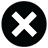# C'mon GirlRed Hot Chili Peppers Lyrics

Everything inside of me
Is burning up for you to see
And if we should get into it
A two-way kind of syndicate
Hold me

Compare us to a Ferris wheel
Just be sure what you want to steal
Some people do it by the book
But I prefer to go by feelTell me

I wanted to get out of here
But every time I reappear
Now I have the words my dear
To whisper out into your ear
Hey, yeah

C'mon girl (let's go)
Let's get it right (let's go)
Let's get it right (let's go)
Let's get it right (let's go)

C'mon girl (let's go)
Let's get it right (let's go)
Let's get it right (let's go)
Let's get it right (let's go)

C'mon girl (let's go)
Let's get it right (let's go)
Let's get it right (let's go)
Let's get it right (let's go)

C'mon girl (let's go)
Let's get it right (let's go)
Let's get it right (let's go)
Let's get it right (let's go)

The spirit of a dragon's tear
Is lovely at this time of year
Is deeper than it will be wide
Hold me

My disenchanted diplomat
Asleep inside the laundromat
Conveyor belts are moving
And I want you to be sure of that
Tell me

And if you let it germinate
I know it will be worth the wait
Disputed by the news that it was
You who tried to instigate
Hey, yeah

C'mon girl (let's go)
Let's get it right (let's go)
Let's get it right (let's go)
Let's get it right (let's go)

C'mon girl (let's go)
Let's get it right (let's go)
Let's get it right (let's go)
Let's get it right (let's go)

C'mon girl (let's go)
Let's get it right (let's go)
Let's get it right (let's go)
Let's get it right (let's go)

C'mon girl (let's go)
Let's get it right (let's go)
Let's get it right (let's go)
Let's get it right (let's go)

Blessed are the hypocrites
Outwitted but she never quits
The trouble with the band of slits
Is washing off the muddy bits
Hey, yeah

C'mon girl (let's go)
Let's get it right (let's go)
Let's get it right (let's go)
Let's get it right (let's go)

C'mon girl (let's go)
Let's get it right (let's go)
Let's get it right (let's go)
Let's get it right (let's go)

C'mon girl (let's go)
Let's get it right (let's go)
Let's get it right (let's go)
Let's get it right (let's go)

C'mon girl (let's go)
Let's get it right (let's go)
Let's get it right (let's go)
Let's get it right (let's go)

Oh yeah she's with me and I'm your man
If I can't find you no one can

Written by: MICHAEL BALZARY, JOHN ANTHONY FRUSCIANTE, ANTHONY KIEDIS, CHAD SMITH

Lyrics Licensed & Provided by LyricFind
To comment on specific lyrics, highlight them